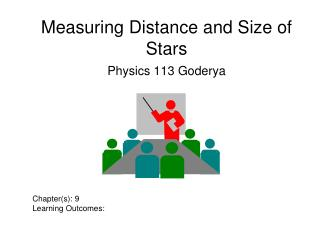Download PresentationMeasuring Distance and Size of Stars

Measuring Distance and Size of Stars - PowerPoint PPT Presentation

Measuring Distance and Size of Stars. Physics 113 Goderya. Chapter(s): 9 Learning Outcomes:. Light as a Wave (1). We already know how to determine a star’s. surface temperature. chemical composition. surface density. In this chapter, we will learn how we can determine its. distance.I am the owner, or an agent authorized to act on behalf of the owner, of the copyrighted work described.
Download PresentationMeasuring Distance and Size of Stars

Download Policy: Content on the Website is provided to you AS IS for your information and personal use and may not be sold / licensed / shared on other websites without getting consent from its author.While downloading, if for some reason you are not able to download a presentation, the publisher may have deleted the file from their server.

- - - - - - - - - - - - - - - - - - - - - - - - - - E N D - - - - - - - - - - - - - - - - - - - - - - - - - -
Presentation Transcript
1. Measuring Distance and Size of Stars Physics 113 Goderya Chapter(s): 9 Learning Outcomes:

2. Light as a Wave (1) We already know how to determine a star’s • surface temperature • chemical composition • surface density In this chapter, we will learn how we can determine its • distance • luminosity • radius • mass and how all the different types of stars make up the big family of stars.

3. Distances to Stars d in parsec (pc) p in arc seconds __ 1 d = p Trigonometric Parallax: Star appears slightly shifted from different positions of the Earth on its orbit 1 pc = 3.26 LY The farther away the star is (larger d), the smaller the parallax angle p.

4. The Trigonometric Parallax Example: Nearest star, a Centauri, has a parallax of p = 0.76 arc seconds d = 1/p = 1.3 pc = 4.3 LY With ground-based telescopes, we can measure parallaxes p ≥ 0.02 arc sec => d ≤ 50 pc This method does not work for stars farther away than 50 pc.

5. Absolute Magnitude To characterize a star’s intrinsic brightness, define Absolute Magnitude (MV): Absolute Magnitude = Magnitude that a star would have if it were at a distance of 10 pc.

6. Absolute Magnitude (2) Back to our example of Betelgeuse and Rigel: Betelgeuse Rigel Difference in absolute magnitudes: 6.8 – 5.5 = 1.3 => Luminosity ratio = (2.512)1.3 = 3.3

7. Intrinsic Brightness/ Absolute Magnitude The more distant a light source is, the fainter it appears.

8. Brightness and Distance (SLIDESHOW MODE ONLY)

9. Intrinsic Brightness / Absolute Magnitude (2) More quantitatively: The flux received from the light is proportional to its intrinsic brightness or luminosity (L) and inversely proportional to the square of the distance (d): L __ F ~ d2 Star A Star B Earth Both stars may appear equally bright, although star A is intrinsically much brighter than star B.

10. Distance and Intrinsic Brightness Example: Recall that: Betelgeuse App. Magn. mV = 0.41 Rigel For a magnitude difference of 0.41 – 0.14 = 0.27, we find an intensity ratio of (2.512)0.27 = 1.28 App. Magn. mV = 0.14

11. Distance and Intrinsic Brightness (2) Rigel is appears 1.28 times brighter than Betelgeuse, Betelgeuse But Rigel is 1.6 times further away than Betelgeuse Thus, Rigel is actually (intrinsically) 1.28*(1.6)2 = 3.3 times brighter than Betelgeuse. Rigel

12. The Distance Modulus If we know a star’s absolute magnitude, we can infer its distance by comparing absolute and apparent magnitudes: Distance Modulus = mV – MV = -5 + 5 log10(d [pc]) Distance in units of parsec Equivalent: d = 10(mV – MV + 5)/5 pc

13. The Size (Radius) of a Star We already know: flux increases with surface temperature (~ T4); hotter stars are brighter. But brightness also increases with size: Star B will be brighter than star A. A B Absolute brightness is proportional to radius squared, L ~ R2. Quantitatively: L = 4 p R2s T4 Surface flux due to a blackbody spectrum Surface area of the star

14. Example: Star Radii Polaris has just about the same spectral type (and thus surface temperature) as our sun, but it is 10,000 times brighter than our sun. Thus, Polaris is 100 times larger than the sun. This causes its luminosity to be 1002 = 10,000 times more than our sun’s.

15. Comparing Size Finding luminosity, temperature and radius of stars in solar units L star / L sun = 4psRstar2 Tstar4 / 4psRsun2 Tsun4 L star / L sun = (Rstar2 / Rsun2)(Tstar4/Tsun4)